# RC Circuits

Electronic circuits often feature a resistor in series with a capacitor. Such circuits are often referred to as resistor-capacitor circuits (or just RC circuits). The effect of the resistor is to limit the rate at which current can flow onto the plates of the capacitor, thereby extending the time required for the capacitor to become fully charged. Once the supply voltage is connected to the circuit, the capacitor begins to charge. During the time in which the capacitor is charging, both the current flowing in the circuit and the voltages across the capacitor and the resistor will be constantly changing. These changing values are referred to as transients. The circuit diagram below shows a simple series connected RC circuit.A series connected RC circuit

When switch S is closed, then according to Kirchhoff's voltage law:

VSUPPLY  =  VC  +  VR

The terminal voltage supplied by the battery VSUPPLY is constant. The capacitor voltage VC is given by Q/C where Q is the charge on the capacitor in coulombs and C is the capacitance value of the capacitor in farads. The voltage drop across the resistor VR is given by I × R, where I is the current flowing in the circuit in amperes, and R is the resistance value of the resistor. The following relationship is therefore true at all times:

 VSUPPLY  =  VC  +  VR  = Q +  I  ×  R C

At the instant when switch S is closed, the circuit will be in its initial state. The charge on the capacitor will be zero, the voltage across the capacitor will be zero, and the voltage drop across the resistor will be equal to the supply voltage. The current I flowing at this point in time will be equal to VSUPPLY/R, since the resistance to current flow is solely due to the resistance R of the resistor. As current continues to flow, the capacitor will become partially charged and the voltage across it will increase as the voltage across the resistor falls. At the same time, the flow of current will slow as the build-up of charge on the plates of the capacitor provides increasing resistance to the movement of electrons. At some period of time after closing the switch, the capacitor will be fully charged and current no longer flows in the circuit. The voltage drop across the resistor will be zero, and the voltage across the capacitor will be equal to the battery terminal voltage VSUPPLY.

The initial rate of change for both the voltage across the circuit components and the current flowing in the circuit is rapid, but the rate of change slows as the capacitor approaches its fully charged state. Graphs showing the changes in VC, VR and I are provided below. The graph showing the way that VC varies with time is called an exponential growth curve, while the graphs depicting the variation of both VR and I over time are called exponential decay curves. The use of the word exponential here indicates that the shape of the curve can be expressed mathematically using an exponential equation.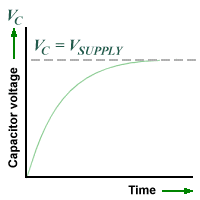The exponential growth curve for capacitor voltageThe exponential decay curve for resistor voltageThe exponential decay curve for current

The diagram below shows a circuit in which a capacitor is charged by a battery when the switch is in position A. When the switch is moved to position B, the charge stored on the capacitor will dissipate and current will flow through the circuit for a time until the capacitor is completely discharged. At the instant when the switch is moved to position B, and providing the capacitor is fully charged, the voltage across the capacitor will be equal to the battery terminal voltage (VSUPPLY). The voltage drop across the resistor will also be equal to VSUPPLY. As current continues to flow, the capacitor will lose charge and the voltage across it will fall, as will both the voltage drop across the resistor and the level of current flowing through the circuit. These transients will decay exponentially, until at some time after moving the switch to position B the capacitor will be fully discharged and current no longer flows in the circuit.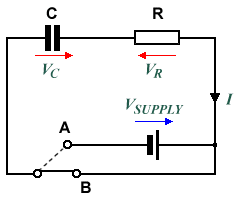The capacitor can be charged and discharged using the switch

Graphs showing the transient curves representing capacitor voltage, resistor voltage and circuit current during capacitor discharge are shown below.Transient curves for voltage and current during capacitor discharge

When a capacitor is charged or discharged through a series connected resistor, the charging or discharging process will take significantly longer than if a resistor is not present. Consider a capacitor with a capacitance C (in farads) charging through a resistor of resistance R (in ohms). It can be shown that, if during charging the charging current remained constant at its initial value, the capacitor would be charged after a time T equal to C × R seconds. In fact, as we can see from looking at the transient curves, the current decreases throughout the charging process. Time T is known as the time constant, and represents the time taken for a capacitor to reach 0.63 (nearly two thirds) of its full charge. In fact, for each further time period T that elapses during charging, the capacitor will increase its charge by 0.63 of the difference in charge between its existing state and the fully charged state.

Once charging starts, the capacitor will be almost (99%+) fully charged after a period equivalent to five time constants (T × 5). The same is true when discharging a capacitor, with the charge on the capacitor falling to 0.37 of its starting value in one time constant. The time constant is a useful measure of how long it takes to charge (or discharge) a capacitor in series with a resistor where both the capacitance of the capacitor and the resistance value of the resistor are known. When discharging the capacitor, the time constant represents the time taken for the capacitor to lose roughly two thirds of its charge. The following graph shows the charge and discharge curves for a capacitor over a period of five time constants.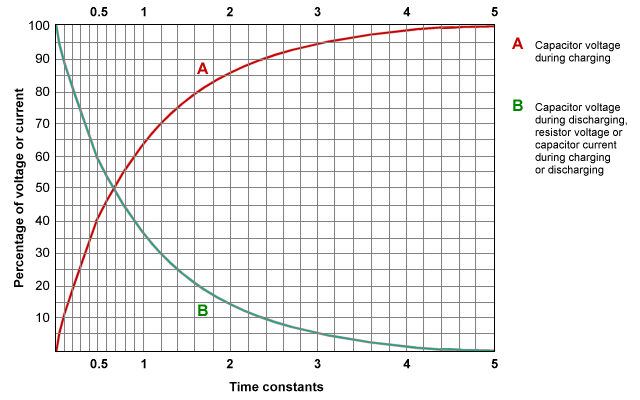Capacitor charge and discharge curves

## Problems

1. Consider the circuit shown below and think about what will happen after the switch is closed.

a. What will be the maximum current through the resistor?

b. What will be the maximum charge on the capacitor?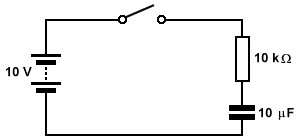2. Examine the circuit shown below. Assume that the capacitor is initially uncharged, with the switch in the position shown on the diagram. The switch will be moved to position A for exactly 5 seconds, then to position B for exactly 5 seconds, then back to its original position. What is the potential difference across capacitor C and the voltage drop across resistor R when:

a. the switch has been in position A for 5 seconds

b. the switch has been in position B for 5 seconds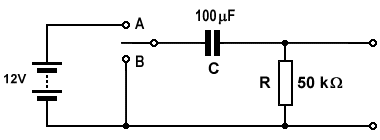3. Complete the time constant table shown below.

CRTime Constant
1μF1MΩ
1kΩ1 second
0.22μF150kΩ
10μF 0.47 seconds

4. A 1.5 V battery is connected to a 1,000 μF capacitor in series with a 150 Ω resistor.

a. What is the maximum current that flows through the resistor during charging?

b. What is the maximum charge on the capacitor?

c. How long does the capacitor take to reach a potential of 1.0 V?